# Geometric Terms

shape term definition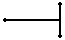Perpendicular Line Segments two line segments which cross to form 90 degree angles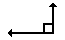Right Angle a 90 degree angle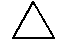Equilateral Triangle a triangle with all sides equal and all angles equal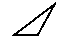Scalene Triangle a triangle having three unequal sides and angles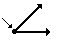Vertex the intersection point of two sides of a plane figure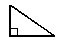Right Triangle a triangle with one internal angle equal to 90 degrees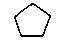Pentagon a polygon with 5 sides and 5 angles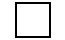Square a rectangle having all four sides of equal length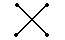Intersecting Line Segments line segments that cross each other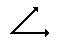Acute Angle an angle less than 90 degrees but greater than 0 degrees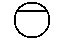Chord the line segment between two points on a given curve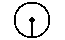Radius a straight line extending from the center of a circle or sphere to the circumference or surface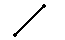Line Segment one part of a line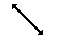Line a continuous extent of length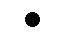Point a position in space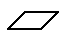Parallelogram a quadrilateral having both pairs of opposite sides parallel to each other
Note: squares and rectangles are also quadrilaterals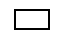Rectangle a parallelogram having four right angles
Note: a square is also a rectangle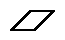Rhombus an equilateral parallelogram having oblique angles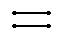Parallel Line Segments line segments that do not intersect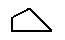Quadrilateral a polygon with four sides
Note: squares, rectangles and trapezoids are also quadrilaterals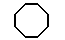Octagon a polygon having eight angles and eight sides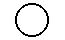Circle a closed plane curve consisting of all points at a given distance from a point within it called the center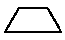Trapezoid a quadrilateral plane figure having two parallel and two nonparallel sides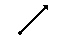Ray the part of a straight line considered as originating at a point on the line and as extending in one direction from that point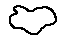Closed Curve a curve that is continuous and has endpoints that meet at the same point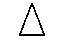Isosceles Triangle a triangle which has two sides equal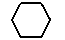Hexagon a polygon having six sides and six angles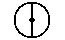Diameter a straight line passing through the center of a circle or sphere and meeting the circumference or surface at each end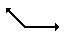Obtuse Angle an angle greater than 90 degrees but less than 180 degrees